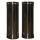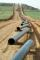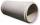# Area + perimeter - math problems

#### Number of problems found: 212

• CylindersThe area of the side of two cylinders is the same rectangle of 33 mm × 18 mm. Which cylinder has a larger volume and by how much?
• AnglesDetermine the interior angles of a rhombus with area 319.1 cm2 and perimeter 72 cm.
• Two squaresTwo squares whose sides are in the ratio 5:2 have a sum of its perimeters 73 cm. Calculate the sum of the area of these two squares.
• ConeCircular cone of height 15 cm and volume 5699 cm3 is at one-third of the height (measured from the bottom) cut by a plane parallel to the base. Calculate the radius and circumference of the circular cut.
• Trapezoid MOThe rectangular trapezoid ABCD with the right angle at point B, |AC| = 12, |CD| = 8, diagonals are perpendicular to each other. Calculate the perimeter and area of ​​the trapezoid.Determine the radius of the circle if its perimeter and area is the same number.
• DescribedCalculate perimeter of the circle described by a triangle with sides 478, 255, 352.
• Right isoscelesCalculate area of the isosceles right triangle which perimeter is 26 cm.
• TinsmithTinsmith construct chimney pipe 145 cm long and 15 cm wide. What are the dimensions of the sheet will have to prepare for the construction of a pipe when the plate overlap at the joint add \$x cm width of the plate?
• FloorThe floor area of ​​the room is 31 m2 and has a width of 4.3 m. How many centimeters of circumference measured the floor on the map at the scale 1:75?
• RectangleIn a rectangle with sides, 8 and 9 mark the diagonal. What is the probability that a randomly selected point within the rectangle is closer to the diagonal than to any side of the rectangle?
• PipelineHow much percent has changed (reduced) area of the pipe cross-section if circular shape changed to square with the same perimeter?
• Arc and segmentCalculate the length of circular arc l, area of the circular arc S1 and area of circular segment S2. Radius of the circle is 11 and corresponding angle is (2)/(12) π.
• GardenHow many steps of 76 cm circumvent square garden with area 1.8 ha?
• TriangleCalculate heights of the triangle ABC if sides of the triangle are a=82, b=44, and c=53.
• CircleHow big is an area of a circle if its circumference is 51.2 cm?
• CapJesters hat is shaped by a rotating cone. Calculate how much paper is needed to the cap 54 cm high when the head circumference is 47 cm.
• Right triangleLegs of the right triangle are in the ratio a:b = 2:8. The hypotenuse has a length of 87 cm. Calculate the perimeter and area of the triangle.
• Isosceles right triangleCalculate the area of an isosceles right triangle whose perimeter is 252 cm.
• Concrete pipeConcrete pipe is cylindrical with an inner diameter 110 cm and outer 120 cm. Calculate the surface of the concrete pipe, if it is 9 m long.

Do you have an exciting math question or word problem that you can't solve? Ask a question or post a math problem, and we can try to solve it.

We will send a solution to your e-mail address. Solved examples are also published here. Please enter the e-mail correctly and check whether you don't have a full mailbox.

Area - math word problems. Perimeter - math word problems.